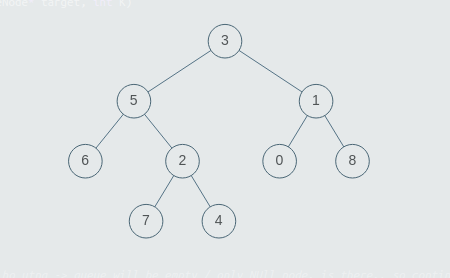New update is available. Click here to update.

# Special Binary Tree.

Last Updated: 4 Dec, 2020
Difficulty: Easy

## PROBLEM STATEMENT

#### Note:

``````1. A binary tree is a tree in which each node can have at most two children.
2. The given tree will be non-empty i.e the number of non-NULL nodes will always be greater than or equal to 1.
3. Multiple nodes in the tree can have the same values, all values in the tree will be positive.
``````
##### Input Format:
``````The first line of the input contains an integer ‘T’, denoting the number of test cases.

The first line of every test case will contain the values of the nodes of the binary tree in the level order form ( -1 for NULL node).  Refer to the example below for further explanation.
``````
##### For Example:
``````Consider the binary tree:
````````````The input for the tree depicted in the above image would be :

3
5 1
6 2 0 8
-1 -1 7 4 -1 -1 -1 -1
-1 -1 -1 -1

Explanation :
Level 1 :
The root node of the tree is 3

Level 2 :
Left child of 3 = 5
Right child of 3 = 1

Level 3 :
Left child of 5 = 6
Right child of 5 = 2
Left child of 1 = 0
Right child of 1 = 8

Level 4 :
Left child of 6 = null (-1)
Right child of 6 = null(-1)
Left child of 2 = 7
Right child of 2 = 4
Left child of 0 = null (-1)
Right child of 0 = null (-1)
Left child of 8 = null (-1)
Right child of 8 = null (-1)

Level 5 :
Left child of 7 = null (-1)
Right child of 7 = null (-1)
Left child of 4 = null (-1)
Right child of 4 = null (-1)

The first not-null node (of the previous level) is treated as the parent of the first two nodes of the current level. The second not-null node (of the previous level) is treated as the parent node for the next two nodes of the current level and so on.

The input ends when all nodes at the last level are null (-1).
``````
##### Output Format :
``````For each test case, print a single line containing “true” or “false”.

The output of each test case will be printed in a separate line.
``````

#### Note :

``````You do not need to print anything, it has already been taken care of. Just implement the given function.
``````

#### Constraints:

``````1 <= T <= 5
1 <= N <= 10 ^ 3
0 <= DATA <= 10 ^ 9

Where ‘DATA’ denotes the value of each node in the given tree and ‘N’ denotes the number of nodes in BinaryTree.

Time limit: 1 sec
``````